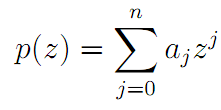The Australian Journal of Mathematical Analysis and Applications

 Home News Editors Volumes RGMIA Subscriptions Authors Contact

ISSN 1449-5910

You searched for frappier
Total of 9 results found in site

4: Paper Source PDF document

Paper's Title:

Asymptotic Inequalities for the Maximum Modulus of the Derivative of a Polynomial

Author(s):

Clément Frappier

Département de Mathématiques et de Génie industriel École Polytechnique de Montréal,
C.P.~6079, succ. Centre-ville Montréal (Québec),

clement.frappier@polymtl.ca.

Abstract:

Letbe an algebraic polynomial of degree ≤n, and let ∥p∥= max {|p(z)|:|z| = 1}. We study the asymptotic behavior of the best possible constant φn,k (R), for k = 0 and k=1, in the inequality ∥p'(Rz)∥ + φn,k (R) |ak| ≤ nRn-1p∥, R → ∞.

3: Paper Source PDF document

Paper's Title:

An Lp Inequality for `Self-Reciprocal' Polynomials. II

Author(s):

M. A. Qazi

Department of Mathematics,
Tuskegee University,
Tuskegee, Alabama 36088
U.S.A.

Abstract:

The main result of this paper is a sharp integral mean inequality for the derivative of a `self-reciprocal' polynomial.

2: Paper Source PDF document

Paper's Title:

Some Inequalities Concerning Derivative and Maximum Modulus of Polynomials

Author(s):

N. K. Govil, A. Liman and W. M. Shah

Department of Mathematics & Statistics,
Auburn University, Auburn,
Alabama 36849-5310,
U.S.A

Department of Mathematics,
National Institute of Technology,
Srinagar, Kashmir,
India - 190006

Department of Mathematics,
Kashmir University,
Srinagar, Kashmir,
India - 190006

Abstract:

In this paper, we prove some compact generalizations of some well-known Bernstein type inequalities concerning the maximum modulus of a polynomial and its derivative in terms of maximum modulus of a polynomial on the unit circle. Besides, an inequality for self-inversive polynomials has also been obtained, which in particular gives some known inequalities for this class of polynomials. All the inequalities obtained are sharp.

Search and serve lasted 0 second(s).